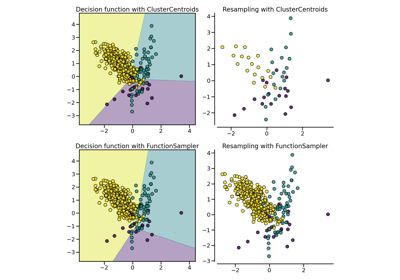# imblearn.under_sampling.NearMiss¶

class imblearn.under_sampling.NearMiss(*, sampling_strategy='auto', version=1, n_neighbors=3, n_neighbors_ver3=3, n_jobs=None)[source]

Class to perform under-sampling based on NearMiss methods.

Read more in the User Guide.

Parameters
sampling_strategyfloat, str, dict, callable, default=’auto’

Sampling information to sample the data set.

• When float, it corresponds to the desired ratio of the number of samples in the minority class over the number of samples in the majority class after resampling. Therefore, the ratio is expressed aswhereis the number of samples in the minority class andis the number of samples in the majority class after resampling.

Warning

float is only available for binary classification. An error is raised for multi-class classification.

• When str, specify the class targeted by the resampling. The number of samples in the different classes will be equalized. Possible choices are:

'majority': resample only the majority class;

'not minority': resample all classes but the minority class;

'not majority': resample all classes but the majority class;

'all': resample all classes;

'auto': equivalent to 'not minority'.

• When dict, the keys correspond to the targeted classes. The values correspond to the desired number of samples for each targeted class.

• When callable, function taking y and returns a dict. The keys correspond to the targeted classes. The values correspond to the desired number of samples for each class.

versionint, default=1

Version of the NearMiss to use. Possible values are 1, 2 or 3.

n_neighborsint or object, default=3

If int, size of the neighbourhood to consider to compute the average distance to the minority point samples. If object, an estimator that inherits from sklearn.neighbors.base.KNeighborsMixin that will be used to find the k_neighbors.

n_neighbors_ver3int or object, default=3

If int, NearMiss-3 algorithm start by a phase of re-sampling. This parameter correspond to the number of neighbours selected create the subset in which the selection will be performed. If object, an estimator that inherits from sklearn.neighbors.base.KNeighborsMixin that will be used to find the k_neighbors.

n_jobsint, default=None

Number of CPU cores used during the cross-validation loop. None means 1 unless in a joblib.parallel_backend context. -1 means using all processors. See Glossary for more details.

RandomUnderSampler

Random undersample the dataset.

InstanceHardnessThreshold

Use of classifier to undersample a dataset.

Notes

The methods are based on .

Supports multi-class resampling.

References

1

I. Mani, I. Zhang. “kNN approach to unbalanced data distributions: a case study involving information extraction,” In Proceedings of workshop on learning from imbalanced datasets, 2003.

Examples

>>> from collections import Counter
>>> from sklearn.datasets import make_classification
>>> from imblearn.under_sampling import NearMiss
>>> X, y = make_classification(n_classes=2, class_sep=2,
... weights=[0.1, 0.9], n_informative=3, n_redundant=1, flip_y=0,
... n_features=20, n_clusters_per_class=1, n_samples=1000, random_state=10)
>>> print('Original dataset shape %s' % Counter(y))
Original dataset shape Counter({1: 900, 0: 100})
>>> nm = NearMiss()
>>> X_res, y_res = nm.fit_resample(X, y)
>>> print('Resampled dataset shape %s' % Counter(y_res))
Resampled dataset shape Counter({0: 100, 1: 100})

Attributes
sample_indices_ndarray of shape (n_new_samples)

Indices of the samples selected.

New in version 0.4.

__init__(self, *, sampling_strategy='auto', version=1, n_neighbors=3, n_neighbors_ver3=3, n_jobs=None)[source]

Initialize self. See help(type(self)) for accurate signature.

## Examples using imblearn.under_sampling.NearMiss¶